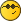Reading order in tableTrond HoyemMember Posts: 116 Threads: 19 Joined: Feb 2017 Reputation: 3 17.11.2019, 16:47 Hi I am working on automatic generation of widgets in the normal visu. So far I have been able to generate the widgets based on a room list and the objects in the object list. It is working rather nicely, but I have one problem. As  I am putting a lot of objects on the widget I have made a table in the script that contains the different parameters for the objects based on the function conde we use in every project. The table look like this for HVAC objects (not yet complete, more codes will be added later): Code:```HVACadresses = {       {order = 'A', kode = 'ACTUAL_TEMP',             statusadr = 'ACTUAL_TEMP',                tekst = 'Temperatur',                                     format = ROverdi,             klasse = 'right-align',                readonly = 1},   {order = 'B', kode = 'DAY_H_SETPOINT',     statusadr = 'DAY_H_SETPOINT',            tekst = 'Varmesetpunkt dag',                        format = RWverdi,                klasse = 'right-align',                readonly = 0},   {order = 'C', kode = 'DAY_C_SETPOINT',     statusadr = 'DAY_C_SETPOINT',            tekst = 'Kjølesetpunkt dag',                        format = RWverdi,                klasse = 'right-align',                readonly = 0},   {order = 'd', kode = 'NIGHT_H_SETPOINT', statusadr = 'NIGHT_H_SETPOINT',        tekst = 'Varmesetpunkt natt',                     format = RWverdi,                klasse = 'right-align',                readonly = 0},   {order = 'e', kode = 'NIGHT_C_SETPOINT', statusadr = 'NIGHT_C_SETPOINT',        tekst = 'Kjølesetpunkt natt',                     format = RWverdi,                klasse = 'right-align',                readonly = 0},   {order = 'f', kode = 'ACTUAL_SETPOINT',     statusadr = 'ACTUAL_SETPOINT',        tekst = 'Faktisk setpunkt',                         format = ROverdi,                klasse = 'right-align',                readonly = 1},   {order = 'g', kode = 'SET_H_OUTP',           statusadr = 'SET_H_OUTP',                tekst = 'Varmepådrag',                                     format = ROverdi,                klasse = 'right-align',                readonly = 1},   {order = 'h', kode = 'SET_H1_OUTP',              statusadr = 'SET_H1_OUTP',              tekst = 'Varmepådrag 1',                                 format = ROverdi,                klasse = 'right-align',                readonly = 1},   {order = 'i', kode = 'SET_H2_OUTP',           statusadr = 'SET_H2_OUTP',                 tekst = 'Varmepådrag 2',                                 format = ROverdi,                klasse = 'right-align',                readonly = 1},   {order = 'j', kode = 'SET_C_OUTP',           statusadr = 'SET_C_OUTP',                tekst = 'Kjølepådrag',                                     format = ROverdi,                klasse = 'right-align',                readonly = 1},   {order = 'k', kode = 'SET_C1_OUTP',              statusadr = 'SET_C1_OUTP',              tekst = 'Kjølepådrag 1',                                 format = ROverdi,                klasse = 'right-align',                readonly = 1},   {order = 'l', kode = 'SET_C2_OUTP',              statusadr = 'SET_C2_OUTP',              tekst = 'Kjølepådrag 2',                                 format = ROverdi,                klasse = 'right-align',                readonly = 1},   {order = 'm', kode = 'ACTUAL_CO2',           statusadr = 'ACTUAL_CO2',                     tekst = 'CO2',                                                     format = ROverdi,                klasse = 'right-align',                readonly = 1},   {order = 'n', kode = 'SETP_CO2',               statusadr = 'SETP_CO2',                         tekst = 'Setpunkt CO2',                                 format = RWverdi,                klasse = 'right-align',                readonly = 0},   {order = 'o', kode = 'SET_CO2_OUTP',         statusadr = 'SET_CO2_OUTP',                tekst = 'Ventilasjonspådrag',                        format = ROverdi,                klasse = 'right-align',                readonly = 1},   {order = 'p', kode = 'SET_V_OUTP',             statusadr = 'SET_V_OUTP',                    tekst = 'Faktisk pådrag til spjeld',        format = ROverdi,                klasse = 'right-align',                readonly = 1}, }``` The problem I am having is that the objects are added to the widget in alphabetical order based on the value in 'kode'. I have tried to change this by adding the column 'order', but that did not help. The first value I use from the table when adding objects are the 'kode' that is used to find the group address from the object list. I thought that the objects would be added in the order I put them in the table as I am using ipairs ro run thought the table and add objects. Anyone has an idea how I can decide the order? There are 10 kinds of people in the world; those who can read binary and those who don'tTrond HoyemMember Posts: 116 Threads: 19 Joined: Feb 2017 Reputation: 3 17.11.2019, 22:45 (This post was last modified: 17.11.2019, 22:45 by Trond Hoyem.) Never mind. I found it. I am looping thru the address list before the table, hence the order of objects in the widget. Will need to change the script a little bit. There are 10 kinds of people in the world; those who can read binary and those who don'tErwin van der ZwartSenior Member Posts: 1440 Threads: 4 Joined: Jul 2015 Reputation: 80 18.11.2019, 21:03 (This post was last modified: 18.11.2019, 21:05 by Erwin van der Zwart.) Hi, You can try this: Code:```tbl = {key2 = 3, key1 = 2, key3 = 1} table.sort(tbl, function(a, b) return a > b end) log(tbl)```BR, Erwin « Next Oldest | Next Newest »